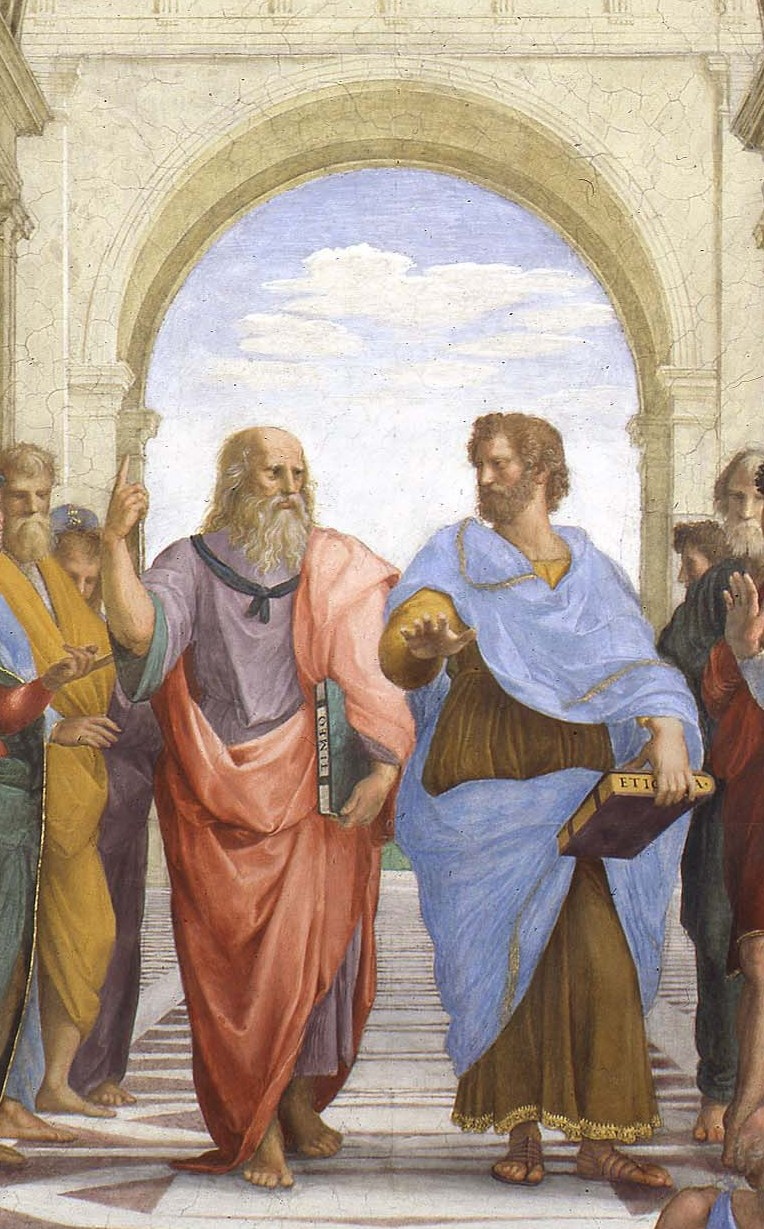## A Dialogue

### 2020-06-03SOCRATES: What is “machine learning”?
PLATO: Why, the improvement of an algorithm’s abilities by the examination of data.
SOCRATES: So, then, a linear regression on a computer is machine learning?
PLATO: Quite so. A candle is not the Sun, but does it not yet illuminate?
SOCRATES: How shall I judge whether the machine has learned?
PLATO: We shall draw some plots.
SOCRATES: What, then, is a plot?
PLATO: Why, a change of coordinates.

``````from sys import stdout

def plot(series, width=80, height=24):
def change_coords(s):
x_min = min(p for p in s)
x_max = max(p for p in s)
y_min = min(p for p in s)
y_max = max(p for p in s)
return [(
int((p - x_min) / (x_max - x_min) * (width - 1)),
int((p - y_min) / (y_max - y_min) * (height - 1))
) for p in s]
to_plot = dict()
for char, s in series.items():
for coord in change_coords(s):
if coord in to_plot:
to_plot[coord] += char
else:
to_plot[coord] = char
for i in range(height):
for j in range(width):
chars = to_plot.get((j, height - 1 - i))
if chars is not None:
if len(chars) > 1:
stdout.write('?')
else:
stdout.write(chars)
else:
stdout.write(' ')
stdout.write('\n')

xs = list(range(10))
ys = [x ** 2 for x in xs]
plot({'o': list(zip(xs, ys))})``````
``````                                                                               o

o

o

o

o

o

o
o
o       o                                                                       ``````

SOCRATES: Quite so. How, then, shall the machine “learn”?
PLATO: By minimizing error.
SOCRATES: And what is error?
PLATO: Why, the difference between what is expected and what is found.
SOCRATES: And how shall we minimize the difference between what is expected and what is found?
PLATO: By rolling down the hill.
SOCRATES: And how do we know which way is “down”?
PLATO: By considering the rate of change.
SOCRATES: What is the rate of change?
PLATO: The rate of change is the ratio of how much this portion of hill changes in elevation to the size of this portion of the hill.

``````def minimize(f, guess, eps=1e-8, rate=1e-3, max_iter=10000):
def approx_f_prime(guess, f_guess):
f_prime = (f(guess + eps) - f_guess) / eps
return f_prime
for _ in range(max_iter):
f_guess = f(guess)
f_prime = approx_f_prime(guess, f_guess)
new_guess = guess - rate * f_prime
if abs(guess - new_guess) < eps:
return new_guess
guess = new_guess
return None

f = lambda x: (x - 2) ** 2
print(minimize(f, 0.0))``````

`1.9999950134026048`

SOCRATES: What shall we learn?
PLATO: Let us find an approximation of a data set by a exponential function y = e^kx.
SOCRATES: Which is to say, let us find the value of k which minimizes the error?
PLATO: Quite.
SOCRATES: So, first, we must define the difference between what is expected and what is found?
PLATO: Exactly so.

``````from math import exp

def model(k, x):
return exp(k * x)

def sse(k, data):
return sum((model(k, x) - y) ** 2 for x, y in data)

NOISY_DATA = [
(1.0, 2.1),
(2.0, 4.6),
(3.0, 9.3),
(4.0, 20.7),
(5.0, 41.4),
]

plot({'o': NOISY_DATA})
print(sse(1.0, NOISY_DATA))
print(sse(0.5, NOISY_DATA))``````
``````                                                                               o

o

o

o
o                                                                               ``````

`12725.389709846757`
`1057.8045226772697`

SOCRATES: I see that you are trying to pull a fast one on me, PLATO. You have taken the square of the difference between that which is expected and that which is found.
PLATO: There is no deception intended—it is to make the hill smoother.
SOCRATES: So that we may roll down it more easily?
PLATO: Quite so.

``````best_k = minimize(lambda k: sse(k, NOISY_DATA), 0.5, rate=1e-5)
print(best_k)``````

SOCRATES: Again I suspect deception—for you have changed the speed with which we roll down the hill.
PLATO: Some hills are steeper than others.
SOCRATES: I remain skeptical. Nonetheless, how shall we judge whether we have learned well?
PLATO: We shall compare that which is expected with that which is found.

``````print(sse(best_k, NOISY_DATA))
plot({'o': NOISY_DATA, 'x': [(x, model(best_k, x)) for x, _ in NOISY_DATA]})``````

`0.9738838331094835`

``````                                                                               ?

?

?

?
?                                                                               ``````

SOCRATES: From the question marks I infer that which is found is about the same as that which is expected, given the change of coordinates.
PLATO: Exactly.
SOCRATES: You have said that for an algorithm to learn is to improve its abilities by the examination of data.
PLATO: That is so.
SOCRATES: You have shown that your algorithm’s abilities have improved at the task of repeating the data it has examined. But what of its abilities to make predictions at new, unexamined values? We do not consider the mockingbird to be the greatest singer among birds.
PLATO: You speak of generalization.
SOCRATES: I speak of utility, for of what value is an algorithm which can be replaced by a mockingbird?
PLATO: That which provides this utility, I call generalization.
SOCRATES: And how may we judge whether an algorithm is general?
PLATO: By testing against that which is known to us, but not examined by the algorithm, and again comparing what is expected to what is found.

``````def leave_one_out(data):
for i in range(len(data)):
train = [d for j, d in enumerate(data) if i != j]
test = [data[i]]

best_k = minimize(lambda k: sse(k, train), 0.5, rate=1e-5)
print(f'k = {best_k}')
print(f'test error = {sse(best_k, test)}')

leave_one_out(NOISY_DATA)``````

`k = 0.7462941022745024`
`test error = 8.407331581956807e-05`
`k = 0.7462671426037844`
`test error = 0.022996313561218864`
`k = 0.7463403623416127`
`test error = 0.0070796292554830996`
`k = 0.7446659508933553`
`test error = 1.078425294824736`
`k = 0.756065110727205`
`test error = 5.90639954833926`

SOCRATES: I see that in each case, we arrive at almost the same model.
PLATO: And thus we believe that the algorithm generalizes.
SOCRATES: This has been most enlightening. Now, may we say that this is “artificial intelligence”?
PLATO: Well…
DIOGENES: Get the hell away from my trash can! What’s a guy got to do to get a little peace and quiet with all these roving philosphers around?

All code is available here.

You can also watch the dialogue in GIF format.

This blog will never track you, collect your data, or serve ads. If you'd like to support the blog, consider our tip jars.

DOGE: `DPZnAPuvYAfCfHZaBjnEkZkdBn4trJbKUK`

BTC: `bc1qjtdl3zdjfelgpru2y3epa56v5l42c6g9qylmjx`

ETH: `0xAf93BaFF53b58D2CA4D2b0F3F5ec79339f6b59F7`